# Difference between revisions of "Generalized derivative"

of function type

An extension of the idea of a derivative to some classes of non-differentiable functions. The first definition is due to S.L. Sobolev (see , ), who arrived at a definition of a generalized derivative from the point of view of his concept of a generalized function.

Letandbe locally integrable functions on an open setin the-dimensional space, that is, Lebesgue integrable on any closed bounded set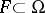. Thenis the generalized derivative ofwith respect to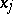on, and one writes, if for any infinitely-differentiable functionwith compact support in(see Function of compact support)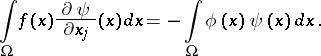(1)

A second, equivalent, definition of the generalized derivative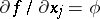is the following. Ifcan be modified on a set of-dimensional measure zero so that the modified function (which will again be denoted by) is locally absolutely continuous with respect tofor almost-all (in the sense of the-dimensional Lebesgue measure)belonging to the projection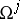ofonto the plane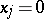, thenhas partial derivative (in the usual sense of the word)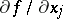almost-everywhere on. If a functionalmost-everywhere on, thenis a generalized derivative ofwith respect toon. Thus, a generalized derivative is defined almost-everywhere on; ifis continuous and the ordinary derivativeis continuous on, then it is also a generalized derivative ofwith respect toon.

Generalized derivatives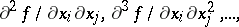of a higher order are defined by induction. They are independent (almost-everywhere) of the order of differentiation.

There is a third equivalent definition of a generalized derivative. Suppose that for each closed bounded set, the functionsand, defined on, have the properties: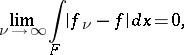and suppose that the functions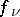,and their partial derivatives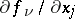are continuous on. Thenis the generalized partial derivative ofwith respect toon(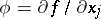) (see also Sobolev space).

From the point of view of the theory of generalized functions, a generalized derivative can be defined as follows: Suppose one is given a functionthat is locally summable on, considered as a generalized function, and letbe the partial derivative in the sense of the theory of generalized functions. Ifrepresents a function that is locally summable on, thenis a generalized derivative (in the first (original) sense).

The concept of a generalized derivative had been considered even earlier (see  for example, where generalized derivatives with integrable square onare considered). Subsequently, many investigators arrived at this concept independently of their predecessors (on this question see ).

How to Cite This Entry:
Generalized derivative. Encyclopedia of Mathematics. URL: http://encyclopediaofmath.org/index.php?title=Generalized_derivative&oldid=16783
This article was adapted from an original article by S.M. Nikol'skii (originator), which appeared in Encyclopedia of Mathematics - ISBN 1402006098. See original article